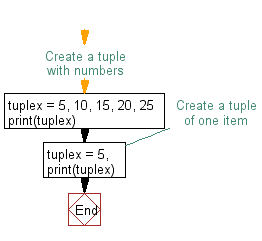﻿ Python: Create a tuple with numbers, and display a member - w3resource# Python Exercise: Create a tuple with numbers, and display a member

## Python tuple: Exercise-3 with Solution

Write a Python program to create a tuple with numbers and print one item.

Sample Solution:-

Python Code:

``````#Create a tuple with numbers
tuplex = 5, 10, 15, 20, 25
print(tuplex)
#Create a tuple of one item
tuplex = 5,
print(tuplex)
```
```

Sample Output:

```(5, 10, 15, 20, 25)
(5,)
```

Flowchart:## Visualize Python code execution:

The following tool visualize what the computer is doing step-by-step as it executes the said program:

Python Code Editor:

Have another way to solve this solution? Contribute your code (and comments) through Disqus.

What is the difficulty level of this exercise?

Test your Python skills with w3resource's quiz

﻿

## Python: Tips of the Day

Returns the maximum value of a list, after mapping each element to a value using the provided function

Example:

```def tips_max(lst, fn):
return max(map(fn, lst))

print(tips_max([{ 'n': 4 }, { 'n': 2 }, { 'n': 8 }, { 'n': 6 }], lambda v : v['n']))
```

Output:

```8
```